### Day 67 - Integration using the Natural Logarithmic Function - 04.23.15

Update
• Unit 5 Test, next Friday!

Questions

Bell Ringer

Review
• Prerequisites
• Derivatives
• Derivative Rules
• Power Rule
• Constant Rule
• Trig Derivatives
• Logarithm Properties
• Differentiation using the Natural Logarithmic Functions (video)

Lesson
• Integration using the Natural  Logarithmic Function (video)
• Checkpoints
• Similar problems are in the book.
• Section 5-2
• Answer Guide is available by the books

Exit Ticket
1. Integration using the Natural Logarithmic Function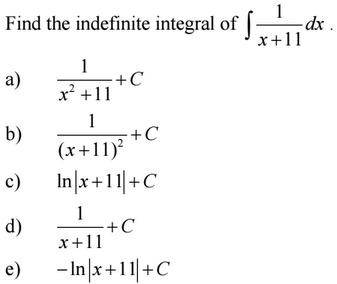1. Integration using the Natural Logarithmic Function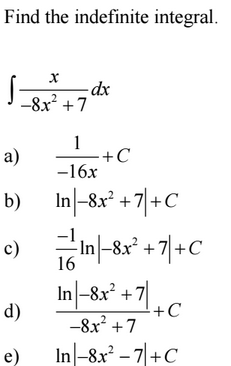1. Integration using the Natural Logarithmic Function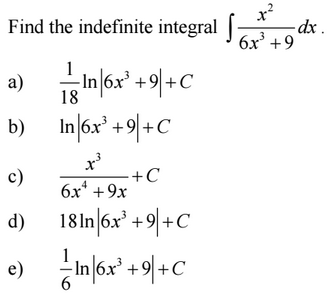1. Integration using the Natural Logarithmic Function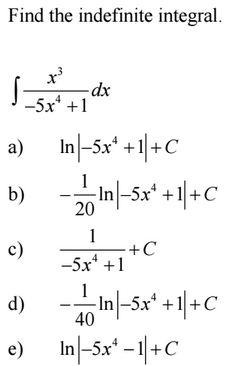1. Integration using the Natural Logarithmic Function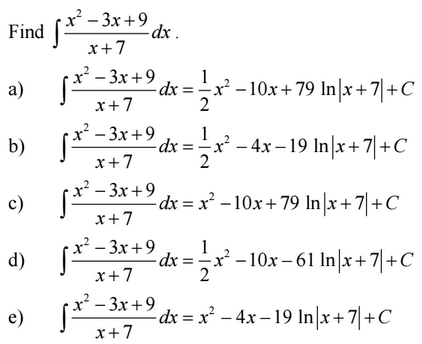Lesson Objective(s)
• How can the derivative of natural logarithmic functions be calculated?
Skills
1. Explain why the power rule does not work when integrating 1/x.
2. Calculate the derivative of the natural logarithmic function.

#### In-Class Help Requests

Standard(s)
• APC.9
• Apply formulas to find derivatives.
• Includes:
• derivatives of algebraic, trigonometric, exponential, logarithmic, and inverse trigonometric functions
• derivations of sums, products, quotients, inverses, and composites (chain rule) of elementary functions
• derivatives of implicitly defined functions
• higher order derivatives of algebraic, trigonometric, exponential, and logarithmic, functions